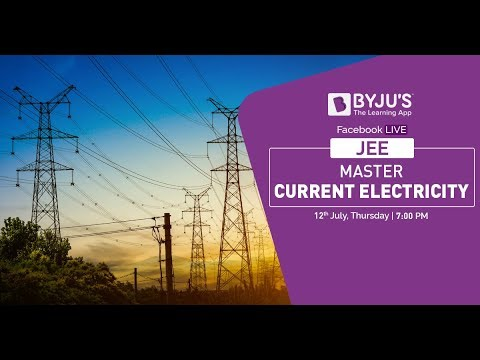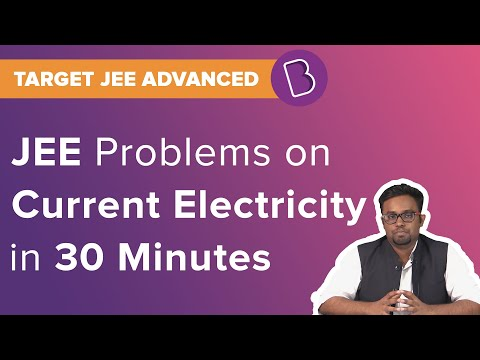# CBSE Class 12 Physics Notes Chapter 3 Current Electricity

## CBSE Class 12 Physics Chapter 3 Notes: Current Electricity

 List of Contents What Is Current? Ohm’s Law Drawbacks of Ohm’s Law What Are Kirchhoff’s Rules? Potentiometer Important Questions

## What Is Current?

Current is referred to the passing of charge through an area per unit of time. For the maintenance of a steady flow of current, it is to be ensured that the circuit is closed and the electric charge is influenced by an external source which moves them from a lower to higher potential energy. The work done to per unit charge to move the charge is known as electromotive force or emf.### Ohm’s Law

According to this law, the electric current flowing through a substance is proportional to the voltage across its ends. In most cases, the carriers of current are the electrons, and the rest types are ionic crystals, negative ions, electrolytic liquids, etc.

### Drawbacks of Ohm’s Law

Every homogeneous conductor containing impurities obeys Ohm’s law, but there are certain drawbacks to it.

• If V depends on I in a non-linear manner, then the law fails.
• The relation between I and V depends upon the sign of V for the same absolute value of V.
• The relation between I and V is non-unique.

### What Are Kirchhoff’s Rules?

Kirchhoff has suggested two rules, which are:

• The Algebraic sum of all the changes in potential around the closed loop is zero.
• In a circuit elements junction, the sum of currents leaving the circuit is equal to the sum of currents entering it.

### Potentiometer

It is a device to compare the potential difference. It can also be used to measure the internal resistance of a cell and in the comparison of two sources

Students can refer to the short notes and MCQ questions along with separate solution pdf of this chapter for quick revision from the links below:

### Important Questions

1. Calculate the maximum current if the emf of a storage battery of a car is 25 V and the battery’s internal resistance is 1 Ω.
2. What is the resistance of the resistor? What is the terminal voltage of the battery when the circuit is closed if a battery of internal resistance 10 Ω and emf 20 V is connected to the resistor and the current in the circuit is 0.2 A?
3. Find the total resistance if three resistors 1 Ω, 2 Ω, and 3 Ω are combined in series.

Related Topics:

CBSE Class 12 Physics Notes

NCERT Solutions for Current Electricity

NCERT Exemplars for Current Electricity

Important Questions for Current Electricity

Current Electricity

## Frequently Asked Questions on Current Electricity

Q1

### A hollow cylinder of length 3 m has inner and outer diameters of 4 mm and 8 mm, respectively. What is the resistance of the cylinder(specific resistance of the material = 2.2 × 10−8 Ωm)?

The resistance of the cylinder, R = 1.75 × 10−3Ω

Q2

### In an experiment with the potentiometer, to measure the internal resistance of a cell. When it is shunted by 5Ω, the null point obtained is at 2 m from one end. When the cell is shunted by 20Ω, the null point is obtained at 3 m from the same end. What is the internal resistance of a cell?

The internal resistance of the cell is 4Ω.

Q3

### The resistance of a wire is 8Ω. It is stretched in such a way that it experiences a longitudinal strain of 400%. What will be the new resistance?

The new resistance will be 200 Ω.